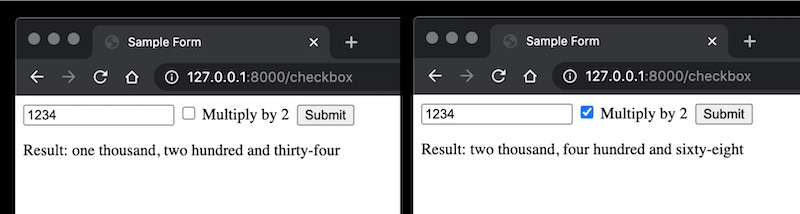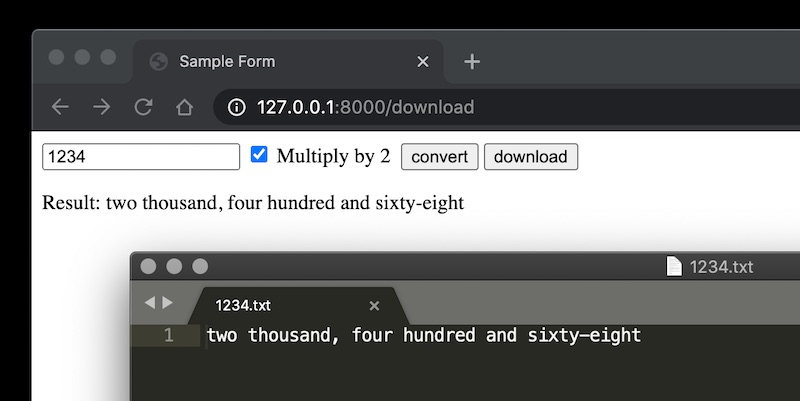In a previous post, I shared about how to build a simple HTML app using `FastAPI`. Here, we’ll extend that app by adding functionality for checkboxes and a download button.

Try it out with the GitHub repo here: `fastapi-html`

## Let’s allow users to alter results

To demonstrate this, we’ll create a checkbox to multiply the input number by two, before spelling it out.

Here’s the `HTML` for it, where we include a new input of type `checkbox` and its label. Note the `name` (`multiply_by_2`) and `value` (`True`) params—we’ll use it later in the post request.

``````<!DOCTYPE html>
<html lang="en">
<meta charset="UTF-8">
<title>Sample Form</title>
<body>
<form method="post">
<input type="number" name="num" value=""/>
<input type="checkbox" id="multiply_by_2" name="multiply_by_2" value="True">
<label for="multiply_by_2">Multiply by 2&nbsp;</label>
<input type="submit">
</form>
<p>Result: </p>
</body>
</html>
``````

We’ll update our post request to take an additional param `multiply_by_2` (from `name`). By default, the value is `False`; but if we check the checkbox, we get the value of `True` (from `value`). This boolean is passed into `spell_number` which handles the logic.

``````@app.post('/checkbox')
def form_post(request: Request, num: int = Form(...), multiply_by_2: bool = Form(False)):
result = spell_number(num, multiply_by_2)
return templates.TemplateResponse('checkbox.html', context={'request': request, 'result': result, 'num': num})
``````

Here are the results with and without checking the option.Results with and without the "Multiply by 2" option

After users view the results, they might want to download it. (In this scenario, it’s a really simple result. Nonetheless, a real app could provide results such as a csv of fraudulent purchases, a pdf report, or an ipython notebook.)

To achieve this, we’ll add a download button. Notice that we now have two inputs of type `submit`. As usual, we’ll use the `name` and `value` params.

``````<!DOCTYPE html>
<html lang="en">
<meta charset="UTF-8">
<title>Sample Form</title>
<body>
<form method="post">
<input type="number" name="num" value=""/>
<input type="checkbox" id="multiply_by_2" name="multiply_by_2" value="True">
<label for="multiply_by_2">Multiply by 2&nbsp;</label>
<input type="submit" name="action" value="convert">
</form>
<p>Result: </p>
</body>
</html>
``````

We’ll update the post request with some basic logic to either return the result via HTML, or to download the file. Note that for `FileResponse` to work, we’ll need to install the `aiofiles` package.

``````@app.post('/download')
def form_post(request: Request, num: int = Form(...),
multiply_by_2: bool = Form(False), action: str = Form(...)):
if action == 'convert':
result = spell_number(num, multiply_by_2)
# Requires aiofiles
result = spell_number(num, multiply_by_2)
filepath = save_to_text(result, num)
return FileResponse(filepath, media_type='application/octet-stream', filename='{}.txt'.format(num))
``````

Here’s how the downloaded file looks like. It is named after the input query `1234.txt`. The results will be the input query spelt out (possibly multiply by two).Try it out with the GitHub repo here: `fastapi-html`

If you found this useful, please cite this write-up as:

or

``````@article{yan2020fastapi2,
author  = {Yan, Ziyou},
journal = {eugeneyan.com},
year    = {2020},
month   = {Aug},